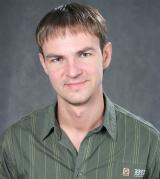## Содержимое страницыДолжность
Научные сотрудники
Информация

Игонин Сергей Александрович

PhD по математике (University of Twente, Нидерланды),

научный сотрудник ЯрГУ им. П.Г. Демидова

Список публикаций

Основные публикации:

1. V.M. Buchstaber, S. Igonin, S. Konstantinou-Rizos, and M.M. Preobrazhenskaia, "Yang--Baxter maps, Darboux transformations, and linear approximations of refactorisation problems", J. Phys. A: Math. Theor. 53 (2020), 504002.

2. S. Igonin, G. Manno, "On Lie algebras responsible for integrability of (1+1)-dimensional scalar evolution PDEs", Journal of Geometry and Physics, 150 (2020), 103596.

3. S. Igonin, G. Manno, “Lie algebras responsible for zero-curvature representations of scalar evolution equations”, Journal of Geometry and Physics, 138 (2019), 297–316.

4. S. Igonin, G. Manno, "On Lie algebras responsible for zero-curvature representations and Backlund transformations of (1+1)-dimensional scalar evolution PDEs". Preprint (2018), https://arxiv.org/abs/1804.04652

5. S. Igonin, G. Manno, "On Lie algebras responsible for zero-curvature representations of multicomponent (1+1)-dimensional evolution PDEs". Preprint (2017), https://arxiv.org/abs/1703.07217

6. G. Berkeley, S. Igonin, “Miura-type transformations for lattice equations and Lie group actions associated with Darboux-Lax representations”, Journal of Physics A: Mathematical and Theoretical, 49 (2016), 275201, 43 pp.

7. S. Igonin, M. Marvan, “On construction of symmetries and recursion operators from zero-curvature representations and the Darboux-Egoroff system”, Journal of Geometry and Physics, 85 (2014), 106–123.

8. S. Igonin, J. van de Leur, G. Manno, V. Trushkov, “Infinite-dimensional prolongation Lie algebras and multicomponent Landau-Lifshitz systems associated with higher genus curves”, Journal of Geometry and Physics, 68 (2013), 1–26.

9. S. Igonin, “Algebras and algebraic curves associated with PDEs and Bäcklund transformations”, Max Planck Institute for Mathematics preprint series, MPIM2010-120 (2010), www.mpim-bonn.mpg.de/preprints/

10. S. Igonin, “Coverings and fundamental algebras for partial differential equations”, Journal of Geometry and Physics, 56 (2006), 939–998.

11. S. A. Igonin, “Miura type transformations and homogeneous spaces”, Journal of Physics A: Mathematical and General, 38 (2005), 4433–4446.

12. S. Igonin, P. Kersten, I. Krasilshchik, “On symmetries and cohomological invariants of equations possessing flat representations”, Differential Geometry and its Applications, 19 (2003), 319–342.

13. S. Igonin, R. Martini, “Prolongation structure of the Krichever-Novikov equation”, Journal of Physics A: Mathematical and General, 35 (2002), 9801–9810.

14. S. Igonin. “Conservation laws for multidimensional systems and related linear algebra problems”, Journal of Physics A: Mathematical and General, 35 (2002), 10607–10617.